# General-type algebraic surface

(diff) ← Older revision | Latest revision (diff) | Newer revision → (diff)

algebraic surface of general type

A surface of one of the broadest classes of algebraic surfaces (cf. Algebraic surface) in the Enriques classification. Namely, a smooth projective surfaceover an algebraically closed fieldis called an algebraic surface of general type if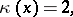where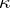is the Kodaira dimension. This condition is equivalent to the fact that for an integer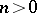the linear system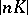, whereis the canonical divisor on, defines a birational mapping ofonto its image in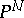for a certain. Every algebraic surface of general type possesses a birational morphism onto its minimal model.

Minimal algebraic surfaces of general type are characterized (see , , ) by each of the following sets of properties:

a)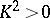andfor any effective divisor;

b)and, where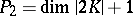is the second plurigenus of;

c)and the surfaceis not rational (cf. Rational surface);

d) there exists an integer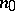such that, for any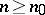, the mappingdefined by the systemis a birational morphism ofonto its image in.

For algebraic surfaces of general type, relations (in the form of inequalities) exist between the numerical characteristics. Letbe the geometric genus and letbe the irregularity of. Then for a minimal algebraic surface of general type the following inequalities hold:

1);

2)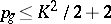ifis even,if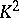is odd (these two inequalities are called Noether's inequalities);

3)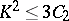, whereis the second Chern class of(or the topological Euler characteristic).

The most complete result on multi-canonical mappingsof algebraic surfaces of general type is the Bombieri–Kodaira theorem: Letbe a minimal algebraic surface of general type over an algebraically closed field of characteristic 0, then the mapping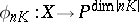is a birational morphism onto its own image for all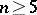. Algebraic surfaces of general type for whichdoes not possess this property exist (see , , ).

How to Cite This Entry:
General-type algebraic surface. Encyclopedia of Mathematics. URL: http://encyclopediaofmath.org/index.php?title=General-type_algebraic_surface&oldid=14784
This article was adapted from an original article by V.A. Iskovskikh (originator), which appeared in Encyclopedia of Mathematics - ISBN 1402006098. See original article